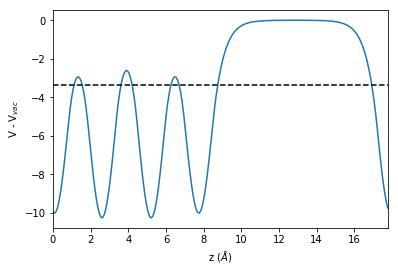# Workfunction of hcp (0001) surfaces¶

In this notebook, we will show how to calculate the workfunction of selected hcp(0001) surfaces using VASP. Please keep in mind that the parameters used here give no converged results. They have been chosen to demonstrate the workflow using inexpensive calculations. For converged results, parameters such as lattice parameters, plane-wave energy cutoffs, reciprocal space sampling or the need to perform spin polarized calculations have to be carefully chosen

In :
import numpy as np
%matplotlib inline
import matplotlib.pylab as plt
import pandas as pd
import time

In :
from pyiron.project import Project

In :
pr = Project("hcp_workfunction")


## Calculating the Workfunction of Mg(0001)¶

### Structure creation¶

We use the create_surface() function which uses the ASE surface generator to build our surface slab structure

In :
 # Now we set-up the Mg (0001) surface
a = 3.1919
c = 5.1852

# Vacuum region to break the periodicity along the z-axis
vac = 10
size = (2, 2, 4)
Mg_0001 = pr.create_surface("Mg",
surface_type="hcp0001",
size=size,
a=a,
c=c,
orthogonal=True,
vacuum=vac)
Mg_0001.plot3d()


### Using selective dynamics¶

We use selective dynamics to restrict relaxation to the surface atoms (first and last Mg layers). We use the advanced array indexing options available in the NumPy package (see here) to detect which atoms are at the surface and then freeze the rest

In :
# Initially freeze all the atoms

# Find which atoms are at the surface
# (based on the z-coordinate)
pos_z = Mg_0001.positions[:, 2]
z_min, z_max = np.min(pos_z), np.max(pos_z)
eps = 1e-4
relax_indices = np.argwhere(((pos_z - eps) > z_min)
& ((pos_z + eps) < z_max ))
relax_indices  = relax_indices.flatten()

# Now allow these atoms to relax

Mg_0001.selective_dynamics[relax_indices] = [True, True, True]


### Setup and execution¶

To automate the calculation we define a function that has as input the project object, structure, job_name, Fermi smearing width, the type of k-point sampling and the plane-wave energy cutoff

In :
def get_ham(proj, basis, name, sigma=0.1, mesh="GP", encut=350):
ham = proj.create_job(pr.job_type.Vasp, name)
ham.set_convergence_precision(electronic_energy=1e-7,
ionic_energy=1e-2)
# Setting fermi-smearing
ham.set_occupancy_smearing(smearing="fermi", width=sigma)
# Ionic_minimization
ham.calc_minimize(ionic_steps=100,
electronic_steps=60,
retain_electrostatic_potential=True,
pressure=None)
ham.structure = basis
ham.set_encut(encut=encut)
if mesh == "GP":
# Only the Gamma point
ham.set_kpoints(scheme="GP")
elif len(mesh) == 3:
ham.set_kpoints(mesh=mesh)
return ham

In :
ham_vasp = get_ham(proj=pr,
basis=Mg_0001,
name="Mg_0001",
sigma=0.1,
mesh="GP",
encut=350)


### Submitting to the queue (optional)¶

If you use a cluster installation of pyiron, you can send the created jobs to the cluster by specifying the name of the queue and the number of cores

In :
# queue = ham_vasp.server.list_queues()[-1]
# ham_vasp.server.queue = queue
# ham_vasp.server.cores = 20


### Choosing an appropriate executable¶

In :
ham_vasp.executable.available_versions

Out:
['5.3',
'5.3_col',
'5.3_col_mpi',
'5.3_mpi',
'5.4',
'5.4.4',
'5.4.4_gam',
'5.4.4_gam_mpi',
'5.4.4_mpi',
'5.4.4_ncl',
'5.4.4_ncl_mpi',
'5.4.4_std',
'5.4.4_std_mpi',
'5.4_gamma',
'5.4_gamma_mpi',
'5.4_mpi']

Since this example uses the $\Gamma$ point only, we can use the VASP Gamma-only version. If you use more k-points choose an appropriate executable

In :
ham_vasp.executable.version = "5.4_gamma"


### Execution¶

The job is ready for execution

In :
ham_vasp.run()


### Post processing¶

To analyze the results we ensure that the job is finished (the if statement in the first line). We then compute the work function by subtracting the Fermi-level from the vacuum level

$\Phi = V_{vac} - \epsilon_F$

In :
if ham_vasp.status.finished:
# Get the electrostatic potential
epot = ham_vasp.get_electrostatic_potential()

# Compute the lateral average along the z-axis (ind=2)
epot_z = epot.get_average_along_axis(ind=2)

# Get the final relaxed structure from the simulation
struct = ham_vasp.get_structure(iteration_step=-1)
r = np.linalg.norm(struct.cell)
z = np.linspace(0, r, len(epot_z))

# Computing the vacuum-level
vac_level = np.max(epot_z)

# Get the electronic structure
es = ham_vasp.get_electronic_structure()
print("wf:", vac_level - es.efermi)
plt.plot(z, epot_z - vac_level)
plt.xlim(0, r)
plt.axhline(es.efermi - vac_level,
color="black",
linestyle="dashed")
plt.xlabel("z ($\AA$)")
plt.ylabel("V - V$_{vac}$");

wf: 3.37343565133## Looping over a series of hcp(0001) surfaces¶

We now repeat the workflow for a set of hcp metals (the chosen lattice parameters are approximate). Note that if you use the same naming convention, pyiron detects that a job with the same name exists ("Mg_0001") and loads the output from this calculation rather than launch a new job with the same name.

In :
hcp_dict = {"Zn": {"a":2.6649, "c": 4.9468},
"Mg": {"a": 3.1919, "c": 5.1852},
"Co": {"a": 2.5071 , "c": 4.0695},
"Ru": {"a": 2.7059 , "c": 4.2815}}

In :
vac = 10
size = (2, 2, 4)
for element, lattice_parameters in hcp_dict.items():
surf = pr.create_surface(element,
surface_type="hcp0001",
size=size,
a=lattice_parameters["a"],
c=lattice_parameters["c"],
orthogonal=True, vacuum=vac)
pos_z = surf.positions[:, 2]
z_min, z_max = np.min(pos_z), np.max(pos_z)
eps = 1e-4
relax_indices = np.argwhere(((pos_z - eps) > z_min)
& ((pos_z + eps) < z_max ))
relax_indices  = relax_indices.flatten()
surf.selective_dynamics[relax_indices] = [True, True, True]
job_name = "{}_0001".format(element)
ham = get_ham(pr, surf,
name=job_name,
sigma=0.1,
mesh="GP",
encut=350)
#ham.server.cores = 20
#ham.server.queue = queue
ham.executable.version = '5.4_gamma'
ham.run()


Now we iterate over all jobs in this project and calculate the workfunction. We also time how long the cell takes to execute

In :
t1 = time.time()
for ham in pr.iter_jobs():
if ham.status.finished:
final_struct = ham.get_structure(iteration_step=-1)
elec_structure = ham.get_electronic_structure()
e_Fermi = elec_structure.efermi
epot = ham.get_electrostatic_potential()
epot_z = epot.get_average_along_axis(ind=2)
vacuum_level = np.max(epot_z)
wf = vacuum_level - e_Fermi
element = final_struct.get_majority_species()[-1]
hcp_dict[element]["work_func"] = wf
t2 = time.time()
print("time: {}s".format(t2-t1))

time: 9.250723838806152s


### Compiling data in a table using pandas¶

In :
df = pd.DataFrame(hcp_dict).T
df = df.rename(columns={'a': 'a [A]',
'c': 'c [A]',
'work_func': 'wf [eV]'})
print(df.round(3))

    a [A]  c [A]  wf [eV]
Co  2.507  4.069    5.569
Mg  3.192  5.185    3.373
Ru  2.706  4.282    5.305
Zn  2.665  4.947    3.603

In [ ]: